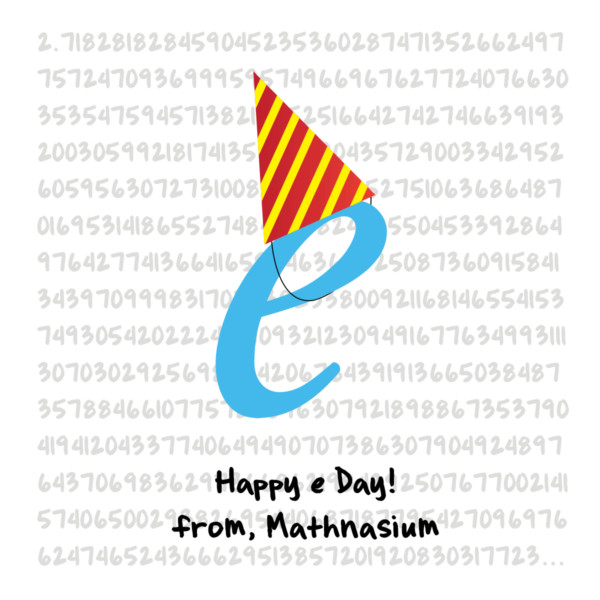# Happy e Day!

By Renee | Added Feb 7, 2015February 7th is e Day! While not as famous as Pi Day, e Day celebrates the mathematical constant e, which has a wide range of mathematical applications. Here's a bit of background on e and why it's worthy of celebration!

As the history of mathematics has unfolded, mathematicians have discovered a number that, in addition to being useful in number theory, occurs over and over in describing nature in mathematical terms.

This number is the mathematical constant e. It is an irrational number (a non–repeating decimal) and also a transcendental number (it is not the solution of any algebraic polynomial). The first 20 decimal places of the numerical value of e are:

### e = 2.71828182845904523536...

Historically, e is sometimes called Euler’s Number after the master Swiss mathematician Leonhard Euler. It is also sometimes called Napier’s Number, in honor of John Napier, the creator of logarithms.

e is one of the most important numbers in mathematics, along with 0 and 1, the additive and multiplicative identities; i, the imaginary unit; π; and ϕ, the number of the Golden Ratio.

Like π, i, and ϕ, e appears unexpectedly in many areas of mathematics.

• e is used to compute Continuous Compound Interest.
• e is used to compute various aspects of Atmospheric Pressure and many other natural phenomena.
• The equations that describe Exponential Growth and Decay involve e.

There are many ways to calculate the value of e. Let's take a look at one way of finding e:Check out this fun song tribute to e from Daniel Wedge!

While e Day might not have the cool factor π Day has, e is definitely worth celebrating. Make sure to wish someone a Happy e Day today!